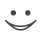This flashcard is just one of a free flashcard set. See all flashcards!

231
11.If a variable x follows the process dx = b dz where dz is a Wiener process, which of the following is the process followed by y = exp(x).
A.dy = by dz
B.dy = 0.5b2y dt+by dz
C.dy = (y+0.5b2y) dt+by dz
D.dy = 0.5b2y dt+b dz